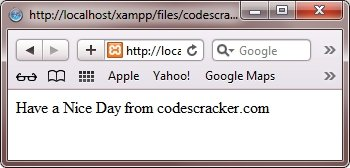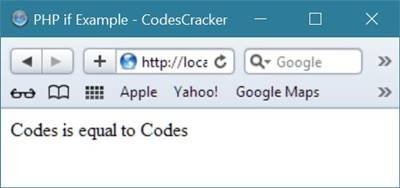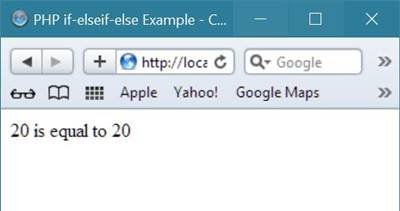# PHP if elseif else Statement

Here you will learn about the if, elseif, and the else statement in PHP with examples.

## PHP if Statement

PHP if statement is used to execute a block of code if the specified condition is true. Here is the general form to use if statement in PHP:

```if(condition)
{
code to be executed here, if the condition evaluates to true;
}```

## PHP if Example

Here is an example uses if statement in PHP. This example will output "Have a Nice Day from CodesCracker.com" if the current time (HOUR) is less than 20:

```<!DOCTYPE html>
<html>
<body>

<?php
\$t = date("H");

if(\$t < "20")
{
echo "Have a Nice Day from codescracker.com";
}
?>

</body>
</html>```

Here is the output produced by the above PHP script:Let's take one more example to understand how if statement works in PHP programming.

```<html>
<title>PHP if Example - CodesCracker</title>
<body>
<?php
\$var1 = "Codes";
\$fname = "Codes";
\$var2 = "Cracker";
\$lname = "Cracker";
\$n1 = 10;
\$n2 = 20;
if(\$var1 == \$fname)
{
echo "Codes is equal to Codes";
}
if(\$var1 == \$var2)
{
echo "Codes is equal to Cracker";
}
if(\$n1>\$n2)
{
echo "10 is greater than 20";
}
?>
</body>
</html>```

As you can see from the above PHP if statement example code, when the condition of the if statement evaluates to be true, then the content inside that if statement will be executed, otherwise the content inside it will be skipped and will not be executed.

Let's take the first if statement that contains

```if(\$var1 == \$fname)
{
echo "Codes is equal to Codes";
}```

As \$var1 contains "Codes" and \$fname also contains "Codes", therefore the above code fragment will become.

```if("Codes" == "Codes")
{
echo "Codes is equal to Codes";
}```

As you can see from the above code fragment, the if statement evaluates to be true, therefore the statement:

`echo "Codes is equal to Codes";`

will be executed and will print Codes is equal to Codes as output.

Here is the sample output produced by the above PHP if statement example.## PHP if-else Statement

PHP if-else statement is similar to the PHP if statement. But in PHP if-else statement, there is an optional else statement available to be executed if the specified condition evaluates to false.

In other word, PHP if-else statement is used executed a block of code (present in the if block) only if the specified condition evaluates to true, otherwise another block of code (present in the else block) will be executed. Here is the general form to use if-else statement in PHP:

```if(condition)
{
code to be executed here, if the condition is true;
}
else
{
code to be executed here, if the condition is false;
}```

## PHP if-else Example

Here is an example, uses if-else statement in PHP. This example will output "Have a Nice Day from CodesCracker.com" if the current time is less than 20, and "Have a Nice Night from CodesCracker.com" otherwise:

```<!DOCTYPE html>
<html>
<body>

<?php
\$t = date("H");

if(\$t < "20")
{
echo "Have a Nice Day from codescracker.com";
}
else
{
echo "Have a Nice Night from codescracker.com";
}
?>

</body>
</html>```

Below is the output of this PHP script:Let's take one more example demonstrating the if-else statement in PHP:

```<html>
<title>PHP if-else Example - CodesCracker</title>
<body>
<?php
\$var1 = "Codes";
\$fname = "Codes";
\$var2 = "Cracker";
\$lname = "Cracker";
\$n1 = 10;
\$n2 = 20;
// first if-else statement
if(\$var1 == \$fname)
{
echo "Codes is equal to Codes";
}
else
{
echo "Codes is not equal to Codes";
}
echo "<br/>";
// second if-else statement
if(\$var1 == \$var2)
{
echo "Codes is equal to Cracker";
}
else
{
echo "Codes is not equal to Cracker";
}
echo "<br/>";
// third if-else statement
if(\$n1>\$n2)
{
echo "10 is greater than 20";
}
else
{
echo "10 is less than 20";
}
?>
</body>
</html>```

As you can see from the program given above, when the condition of the if statement evaluates to be true, then the content inside it will be executed, otherwise the content present inside the else statement of that if statement will be executed.

Here is the sample output produced by the above if-else statement example code in PHP## PHP if-elseif-else Statement

PHP if-elseif-else statement is used to perform some action based on more than one condition. You can use PHP if-elseif-else statement to specify a new condition to be tested, if the first condition evaluates to be false. Here is the general form of PHP if-elseif-else statement:

```if(condition1)
{
code to be executed here, if condition1 is true;
}
elseif(condition1)
{
code to be executed here, if condition2 is true;
}
else
{
code to be executed here, if both the condition is false;
}```

## PHP if-elseif-else Example

Here is an example uses PHP if-elseif-else statement. This example example will output "Have a Nice Morning from CodesCracker.com" if the current time is less than 10, and "Have a Nice Day from CodesCracker.com" if the current time is less than 20. Otherwise it will output "Have a Nice Night from CodesCracker.com"

```<!DOCTYPE html>
<html>
<body>

<?php
\$t = date("H");
if(\$t < "10")
{
echo "Have a Nice Morning from codescracker.com";
}
elseif(\$t < "20")
{
echo "Have a Nice Day from codescracker.com";
}
else
{
echo "Have a Nice Night from codescracker.com";
}
?>

</body>
</html>```

It will display the following resultHere is another example on if-elseif-else statement in PHP.

```<html>
<title>PHP if-elseif-else Example - CodesCracker</title>
<body>
<?php
\$n1 = 20;
\$n2 = 30;
\$n3 = 40;
\$n4 = 20;
if(\$n1>\$n2)
{
echo "20 is greater than 30";
}
elseif(\$n1>\$n3)
{
echo "20 is greater than 40";
}
elseif(\$n1==\$n4)
{
echo "20 is equal to 20";
}
elseif(\$n1>\$n4)
{
echo "20 is greater than 20";
}
else
{
echo "none";
}
?>
</body>
</html>```

As you can see from the above PHP example of if-elseif-else statement, if the condition of if statement evaluates to be true, then the content inside it will be executed and all the next elseif and else statement will be skipped, and if the condition of the if statement evaluates to be false, then the program control goes to the elseif statement, and check if its condition evaluates to be true, if yes, then the content inside that elseif will be executed and the next all statement will be skipped, this control goes until the last conditional statement, that is, else.

In case, if none of the if, or elseif statement's condition evaluates to be true, then the content inside the else statement will be executed.

Here is the sample output produced by the above if-elseif-else statement in PHP.Tools
Calculator